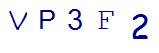# 3 Common Challenges Students Face with 8th Grade Algebra ProblemsLast Updated on May 26, 2022 by Thinkster

For some students, algebra is a very challenging topic. If you find that your child is struggling with 8th-grade algebra problems, here are three areas you should look at improving.## 1. Strengthen Foundational Math Skills

Algebra is about taking the information you already know about numbers, expanding it, and then applying it. Students who do not have a solid foundation in addition, subtraction, multiplication, division, decimals, and fractions are going to struggle when they reach 8th-grade Algebra. Building and improving those foundational skills can help set your child up for greater success.

## 2. Check Your Child’s Understanding for Solving Basic Equations

Solving equations is the core of 8th-grade algebra. Students start by learning to solve simple linear equations with just one variable, such as 35 = 4x + 7. If students have not had a solid foundation in learning how to solve equations, then this sort of equation may be challenging for them.

The key to teaching your child how to solve equations is to think of the problem like a balance: each side has to remain balanced and the equal sign is the pivot. In order to determine what the variable is, you need to add, subtract, multiply or divide to “get rid of” the numbers surrounding the variable. However, because it is a balance, whatever you do to one side of the equal sign, you have to do to the other.

So, in the example 35 = 4x + 7, you would begin by subtracting the seven from both sides of the equal sign. This gives you 28 = 4x. To solve for x, you then need to divide both sides by 4.

## 3. Check That Your Child Can Use the Distributive Property

Once your child understands how to solve linear equations, they will face more complex algebra problems. A student may, for example, have to solve:

-9 – (9x -6) = 3 (4x +6)

In order to begin solving this problem, the student must simplify it by using the distributive property.The student “distributes” the negative sign in front of the (9x – 6) and the 3 in front of the (4x + 6).

Distribution involves multiplying everything inside the parenthesis by the coefficient. In the case of the – sign, the coefficient is – 1. Now the problem becomes -9 – 9x + 6 = 12x + 18. The student combines the -9 and + 6 to yield -9x – 3 = 12x + 18, and the problem is solved as a simpler algebraic expression.

Failure to understand and properly apply the distributive property from the start will make this algebra problem seem very difficult. This is why understanding certain skills and strategies is so important for algebra.

## Join a Digital Math Tutoring Program to Help with 8th Grade Algebra Problems

Have you noticed your child struggling with 8th-grade algebra problems? There are different math help solutions available, and a digital math tutoring program, like Thinkster Math, can help your child improve their algebraic skills.

With Thinkster, your child gets a certified coach right in the comfort of your own home. Whether your child needs extra help with foundational math skills that were taught before 8th grade or current algebra problems, Thinkster can help conveniently and effectively.

SummaryArticle Name
3 Common Challenges Students Have with 8th Grade Algebra
Description
Is your child struggling with 8th grade algebra? Here are some common causes that might be leading to this, and how you can overcome them.
Author
Publisher Name
Thinkster Math
Publisher Logo

## Subscribe to Thinkster Blog## Recommended Articles### The 3 Core Principles of Accelerated Learning in K-8 Math

In my quest to create a failure-proof system for learning math, I discovered 3 core principles that shorten...